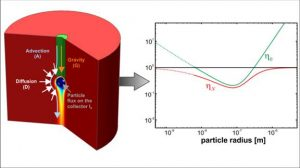ETAMMS2015 is a spreadsheet that lists the numerical data used in the study “An extended and total flux normalized correlation equation for predicting single-collector efficiency” (these data can be downloaded at this link).

In this study a novel total flux normalized correlation equation ηN_MMS is proposed for predicting single-collector efficiency under a broad range of parameters accounting, for mutual interaction of concomitant transport mechanisms (i.e. advection, gravity and Brownian motion) and for the finite size of particles (steric effect). The correlation equation is based on a combination of Eulerian and Lagrangian simulations performed in a geometry consisting in a sphere enveloped by a cylindrical control volume. The correlation equation was also extended to include porosity dependence and a reduced form was proposed by elimination of the less relevant terms (for further information please refer to the full paper and the SI).

The spreadsheet available at this link reports the numerical simulation data, divided according to the active transport mechanisms.

Moreover, two worksheets are available to evaluate a particular efficiency value and to plot efficiency trend versus particle size. In particular, the full and reduced models are implemented for high porosity values (first worksheet) and for realistic porosity values (second worksheet).### References

Messina F., Marchisio D., Sethi R. (2015) An extended and total flux normalized correlation equation for predicting single-collector efficiency, Journal of Colloid And Interface Science, 10.1016/j.jcis.2015.01.02 pdf